## Saturday, August 31, 2013

### New Comics Coming in September...

Yes, there will be new comics coming in September, and I don't mean the third week of September. (At least, I hope I don't.) And if not new math comics, then new math content should show up soon.

I'll keep this brief: a few weeks ago, my Mom had a stroke. A week ago, she passed away. I've spent a lot of time at the hospital, sitting quietly, waiting, sometimes talking, wondering if she heard me, sometimes just reading quietly. It was a little like my Mothers Day 2013 comic, except, at that time, she was just recuperating from, let's just say, illnesses related to age.

I have wanted to keep my mind occupied, but regular comics just weren't going to happen. I'd like to have a couple done before I start posting them again, rather than proceeding if fits and starts. I need to move on and keep doing what I was doing and what I like doing.

Mom would have wanted me to do that.

## Thursday, August 22, 2013

### (Still on Hiatus:) Burke's Laws

(Click on the cartoon to see the full image.)

I'm still "on hiatus", but I'm also need a little bit of a release.
The comic below features a couple of "laws" I first spouted at some point back in the mid-80s, between college and career. The pictures are new. Enjoy.

Come to think of it, in some cases, playing games and looking at naughty pictures have become the same thing, too.

I've voiced a few "Laws" like these over the years, but these are two that always stuck with me, and both, so far, are still true.

Whether or not they are actually "laws" or "theories" or "postulates" or anything else, I don't know. "Burke's Law" always sounded cool to me, and that was before I even knew it was the title of an old TV show.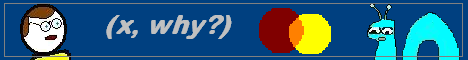## Monday, August 19, 2013

So the new attempt by spammers to get you to their site isn't to put links in comments, which I'll delete as soon as I see them. Now, they're registering for blogger IDs, and leaving three-word comments about how "great" or "cool"

Not much I can do about it, but be warned: Do NOT click on the their usernames, because you will open yourself up to spam.

Ifyou are a real human and wish to comment, and I wish you would, please, leave a sentence or two, so I know you are real. Thanks.

## Saturday, August 17, 2013

### Integrated Algebra Regents Exam (August 2013) Discussion, Part II, III and IV

EDIT: Welcome to my website. If you like the information in this blog entry or find it useful, please, feel free to leave a comment. Thank you for visiting.

### Part II

31. A jogger ran at a rate of 5.4 miles per hour. Find the jogger's exact rate, in feet per minute. Note: “1 mile = 5280 feet” was given.

To convert from miles per hour to feet per minute, you need to multiply by 5280 feet/minute and 1 hour/60 min. Why is the 60 in the bottom? Look at this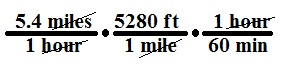The hour units cancel as do the mile units, leaving only feet per minute. Evaluate the expression and you get 475.2 feet per minute.

Partial credit for doing one step, or miscalculating or for rounding to the nearest foot.

32. Simplify twice the square root of 108, which I’ll write as 2(108)^.5
A reminder: “^.5” means “raised to the one-half power”, which means to take the square root. You can use this form in spreadsheets.

The largest perfect square that is a factor of 108 is 36. So you have (2)(36*3)^.5, which is (2)(6)(3)^.5, which is 12(3)^.5, or 12 times the square root of 3.

Partial credit if you didn't simplify completely, or if you gave the decimal equivalent (which in my opinion shouldn't be worth anything, but, as it has been noted, I don't write the exams).

33. Adrianne invested \$2000 in an account at a 3.5% interest rate compounded annually. She made no deposits or withdrawals on the account for 4 years. Determine, to the nearest dollar, the balance in the account after the 4 years.

Compounded interest. (2000)(1.035)4 = 2295.046, which is \$2295 to the nearest dollar.

I don't know if there's credit for calculating simple interest. A point is lost for not rounding correctly, which on a two-point question hurts a lot!

### Part III

34. Miller's Department Store is having a sale with a 25% discount on mattresses. If the sales tax rate is 8%, how much change will Frank receive from \$800 if he purchases a mattress regularly priced at \$895 during this sale?

In one line (but don't do this in one line), it's:

800 - (895 * .75)(1.08) = 800 - 724.95 = 75.05

Find 75% of \$895 (or find 25% of it and then subtract that amount from 895) and multiply by 1.08 (or find 8% of the subtotal and then ADD that to the subtotal) to get the total amount spent. Subtract that from \$800 to find out how much change he'll get.

Partial credit if you stopped along the way or made a calculation error.

35. The difference between two numbers is 28. The larger number is 8 less than twice the smaller number. Find both numbers. Let x be the smaller number and y be the bigger number. Then the two equations that you need are

y - x = 28
y = 2x - 8
Now, this is a good case for substitution (unlike question 15).
2x - 8 - x = 28
x - 8 = 28
x = 36 (the smaller number)
so y = 36 + 28 = 64 or y = 2(36) - 8 = 72 - 8 = 64. (check!)

Partial credit for only finding x and forgetting about y. Checking isn't required, but it's a good idea. If you got the wrong x, but evaluated y using that x, that's consistency, so it's partial credit.

36. Janis measures the dimensions of the floor in her rectangular classroom for a rug. Her measurements are 10.50 by 12.25 feet. The actual measurements of the floor are 10.75 feet by 12.50 feet. Determine the relative error in calculating the area, to the nearest thousandth.

Hey, tutoring class! We did a similar problem, only with Volume. This one is an easier example of this type of question compared to what they usually ask. Make sure you round correctly.

Find the two areas. Then calculate (the difference of the areas)/(the actual area).

(134.375 - 128.625)
134.375
= 0.042790..., which is 0.043 to the thousandth place.

The two areas together are worth a single point. The calculations give you the next point. The final answer, rounded correctly, give you the third point.

37. A graph. Absolutely no reason that you should lose any points if you plot the graph correctly, label the lines and state the solutions (the points of intersection).

And if you realize that y + 1 = -2x is the same as y = -2x - 1, you can put both equations into your graphing calculator, graph it and look at the Table of Values. All you would need to do is copy the points onto your paper and draw the lines. They meet at (-2, 3), which must be labeled.

If you graphed a parabola with an error or an incorrect line (ex, y = -2x + 1), the lines should still intersect and you can state (approximately, if necessary) the point or points of intersection. If your graph is so far off that there are no points of intersection, then you would have written "No solution.", although that should have tipped you off that there's a mistake.

38. It's easier to use a picture than to explain: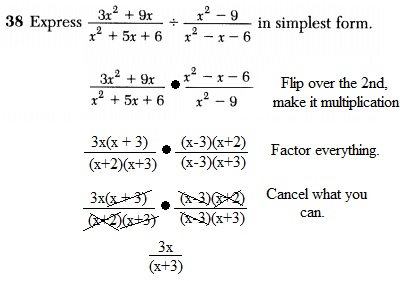No points if you canceled out all the x2's.

39. A bottle contains 12 red marbles and 8 blue marbles. A marble is chosen at random and not replaced. Then, a second marble is chosen at random.
a. Determine the probability that the two marbles are not the same color.

You need to find P(red then blue) and P(blue then red), and add them. (They'll be the same.)
so (12/20 * 8/19) + (8/20 * 12/19) = 192/380

b. Determine the probability that at least one of the marbles is red. You can find P(Red then blue) and P(blue then red) -- which we just did -- and P(two reds) and add them.
So (192/380) + (12/20 * 11/19) = (192/380) + (132/380) = 324/380

Alternatively, you could find the probability that both are blue and then subtract that from 1:
1 - (8/20 * 7/19) = 1 - (56/380) = (380/380) - (56/380) = 324/380.

## Friday, August 16, 2013

### Integrated Algebra Regents Exam (August 2013) Discussion, Part 1

I've been itching to do this for a few days now, just to get my mind off other things.

EDIT: I've added the questions to the answers listed below because students are using search engines to find the answers, and that will lead them here!

### Part 1: Multiple Choice

As there are 30 questions, I will be brief about them. The questions and answers are online, so I'm restrict my comments to the "tricky" ones. I won't retype the entire type or restate what is (hopefully) obvious.

1. Which situation describes a negative correlation?
Amount of gas used and the amount of gas left make a negative correlation. The others are positive.

2. The sum of 8n - 3n + 10 and -3n2 - 6n - 7 is
Watch the signs. Choice (1).

3. Which event is certain to happen?
Certainty in probability means 100% of the time. As much as I'd like to say "The Yankees" (but that would be boring), it's "the sun rises in the east."

4. Noj is 5 years older than Jacob. The product of their ages is 84. How old is Noj?
(x)(x+5)=84. The older brother is 12. (3)
You don't have to solve a quadratic equation to get this. You can look at the choices. Multiply each number by the number that is five less. Which product is 84? (6)(1), (7)(2), (12)(7) or (14)(9)?

5. Marie currently has a collection of 58 stamps. If she buys s stamps each week for w weeks, which expression represents the total number of stamps she will have?
Seriously, this question looks recycled. Not "similar", I mean "recycled". 58 + sw.

6. Given: A  {all odd integers from 1 through 19, inclusive} B  {9, 11, 13, 15, 17} What is the complement of set B within set A?
They want the members of A that are NOT in B. "Inclusive" means that 1 and 19 are elements of set A. Answer is (4).

7. Which equation represents a line that is parallel to the line whose equation is y = - 3x - 7?
This is a "gimme". Parallel lines have the same slope. You didn't even have to find the slope because the question and the choices were all in slope-intercept form.

8. Which graph does not represent the graph of a function?
Choice (3) fails the Vertical Line Test and is not a function. The others are linear, quadratic and absolute value functions.

9. Which value of x is in the solution set of -3x + 8 ≥ 14?
Plug in the choices one at a time and the answer is the first one. In an inequality such as this one, the answer almost always has to be the highest or the lowest number. It's the lowest.

10. What is the slope of the line that passes through the points (4,-7) and (9,1)?
Slope of a line = rise / run or "change in y" / "change in x". (2) 8/5

11. Simplify the fractions by cancelling common factors. (3) and (4) aren't simplest form, and there are more factors of x in the denominator than in the numerator, so it's choice (2).

12. The box-and-whisker plot below represents a set of grades in a college statistics class. [GRAPH OMITTED] Which interval contains exactly 50% of the grades?
My tutoring class just went over box-and-whisker plots (see the comic on Quartiles), so I hope they all got this one. Each section represent 25% of the data, so two sections is 50%. (4) includes exactly two quartiles.

13. An art studio has a list of information posted with each sculpture that is for sale. Each entry in the list could be classified as quantitative except for the
"Quantitative" is an answer to "how much" or "how many". (It's numeric.) The name of the artist is not quantitative. (Note: Remember, not all numeric data is quantitative, but that's for another day.)

14. Which graph represents the inequality y ≥ x + 3?
">" means greater than or equal to. Solid line and shaded above. (2)

15. Using the substitution method, Ken solves the following system of equations algebraically
2x - y = 5
3x + 2y = - 3
Which equivalent equation could Ken use?

Okay, this one is just EVIL! When given a choice, it's easier to solve a problem like this through elimination. I don't force my students to use substitution when a different method is better and simpler. In problem solving you are supposed to come up with a plan of action. This goes against the plan.

Anyway . . . rewrite 2x - y = 5 as 2x - 5 = y and substitute and you get 3x + 2(2x - 5) = -3, which is (1).

16. A fraction is "undefined" when the denominator is zero, so the real question here is "Solve for x when x2 - 2x - 15 = 0. That happens when x = 5 or x = -3. The correct choice is (4). (Again, you could have substituted for each of the choices, which may have been faster for you.)

17. The statement |-15| < x < |-20| is true when x is equal to
Definition of absolute value. Notice that x does not have absolute value symbols, so the only correct answer is (3).

18. Which equation is true?
A little involved, but easily done. You have evaluate the left side to see if it equals the right side. When dividing fractions you flip the second fraction over and multiply. c5 * c is not equal to c4, so (1) is wrong. Choice (2) applies the exponent to everything inside the parentheses, so (2) is the answer. In (3), (t8/t5)2 would have an exponent of 6 not 5. In (4), the product of the co-efficients (-2)(3) is (-6), not 1 (which is omitted).

19. The equation 3(4x)  (4x)3 illustrates which property?
Switching the order of two multiplicands is commutative.

20. Monique has three sons who play football, two sons who play baseball, and one son who plays both sports. If all of her sons play baseball or football, how many sons does she have?
I hate the wording of this problem because the word "only" might be inferred by students reading it, and they would be incorrect for doing that. One son plays both sports, so one son just plays baseball, and two just play just football, so she has four sons. (4)

21. Written in set-builder notation, S = {1, 3, 5, 7, 9} is
Even if you didn't know what set-builder notation was, you could infer the correct answer by knowing that "1 < x < 9, where x is an odd number" means "1, 3, 5, 7, 9".

22. Which is the equation of a parabola that has the same vertex as the parabola represented by y = x2, but is wider?
To widen a parabola, you need a smaller (in this case, fractional) co-efficient. If you change the y-intercept, you will change the vertex. Choice (4).

23. In right triangle ABC, m∠C = 90, AC = 7, and AB = 13. What is the length of BC?
Since angle C is the right angle, side AB is the hypotenuse. The length of BC is the square root of (132 - 72 = 169 - 49 = 120). Choice (3). If you added, you incorrectly got (4) as your answer.

24. A cube, with faces numbered 1 to 6, is rolled, and a penny is tossed at the same time. How many elements in the sample space consist of an even number and a tail?
Three elements: 2T, 4T, 6T. I hope you did NOT try using the Counting Principle on this one.

25. If the volume of a cube is 8 cubic centimeters, what is its surface area, in square centimeters?
Volume of a cube is s3 = 8, so s = 2. The surface area is 6s2, which is 24. (2) Fixed

26. A designer created a garden, as shown in the diagram below. The garden consists of four quarter-circles of equal size inside a square. The designer put a fence around both the inside and the outside of the garden.

Which expression represents the amount of fencing, in yards, that the designer used for the fence.
Four quarter-circles is one full circle. The circumference of the circle is 10pi (not 5pi or 25pi). The perimeter of the square is (4)(10) = 40. Answer (1) 40 + 10pi.

27. Mr. Taylor raised all his students’ scores on a recent test by five points. How were the mean and the range of the scores affected?
Curveball! They included the range. I went over this question with my students, but I didn't include the range (which wasn't included in previous tests). The mean goes up by five because the all the scores went up by five; however, the range remains the same because the highest score and the lowest score were increased by the same amount.

28. The common denominator between 3a and 2a is 6a. Multiply the first fraction by (2/2) and the second by (3/3) and you get (8/6a) - (15/6a), which is (-7/6a). Choice (3). No exponents!

29. Which ratio represents the cosine of angle A in the right triangle below?
What did I tell everyone about their overuse of 3-4-5 triangles? Cosine is adjacent/hypotenuse, 3/5. (1). Cosine can't be greater than 1, so (2) and (4) are right out.

30. If 2y + 2w = x, then w, in terms of x and y, is equal to
Subtract 2y from both sides and then divide by 2. You get (x - 2y)/2. (2)

How did you do? Be honest. Fifteen correct answers is a passing grade and everything else is extra, but we're all hoping for a lot better than merely passing, right?

Parts II, III and IV to come.

## Tuesday, August 13, 2013

### Algebra and Geometry Regents Discussion, Anyone?

I'm not in school and didn't (won't) see the exams, but if anyone would like to discuss any questions, I'd be happy for the diversion.

I don't know when the exams will be posted online for all to see, so please describe any problem you have a question or complaint about.

Thanks.

UPDATE 8/14/13: I still haven't seen the test but they posted the Conversion Chart. As usual, a Raw Score of 30 out of 87 (just over one-third) curved to a passing grade of 65.

To those who made that, Congratulations, and please don't take this the wrong way when I put out that passing a test with only one-third of the answers correct is NUTS. But that's the system we have and it isn't going anywhere.

The Geometry Chart is out as well. A Raw Score of 41 out of 86 (not quite half) merited a final score of 65.

UPDATE 8/17/13:There are two new threads dealing with the Algebra Regents. The Geometry thread is coming soon.
Part 1, multiple choice, and Parts 2, 3 and 4, Open ended.

## Monday, August 12, 2013

### Brief Hiatus: a few days?

There are some family issues going on right now which will take up most of my time for the next few days. Be back soon.

## Friday, August 09, 2013

### Quartiles

(Click on the cartoon to see the full image.)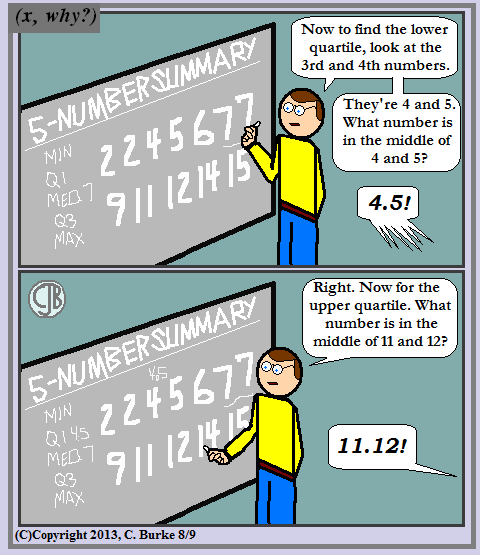To be fair, only one student shouted that. I pretended not to hear it.## Wednesday, August 07, 2013

### If I Wrote the State Exams . . .

(Click on the cartoon to see the full image.)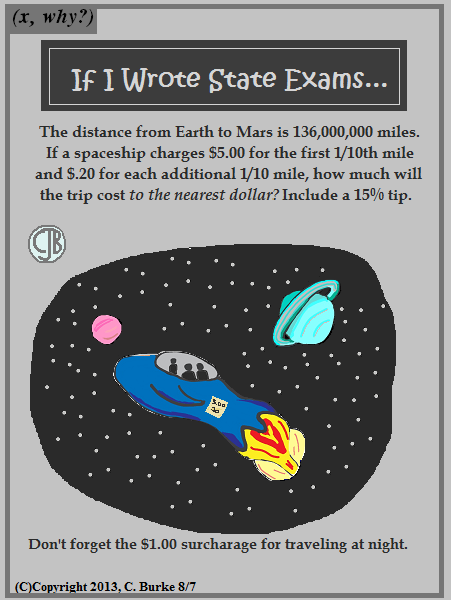Alternate Title: 'Why I'm Not Allowed to Write State Exams'## Monday, August 05, 2013

### Lost in Thought

(Click on the cartoon to see the full image.)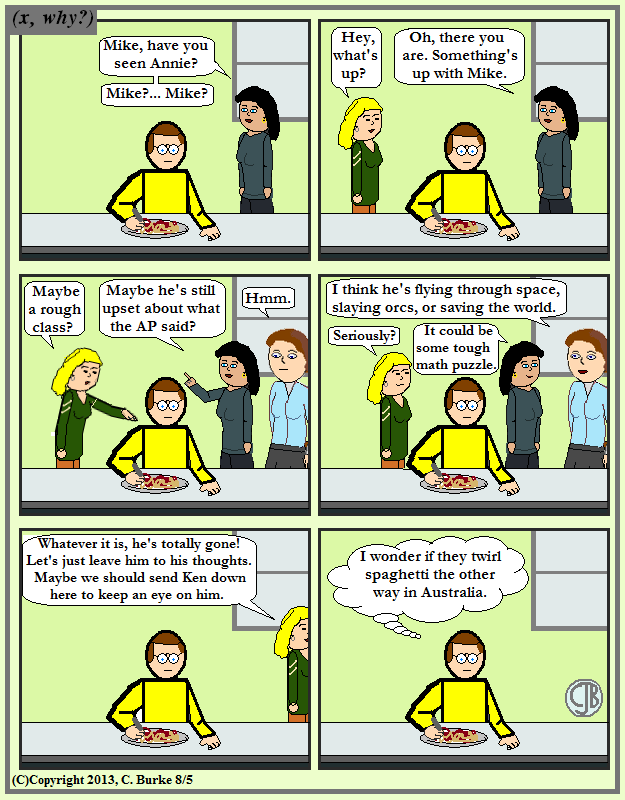The scary part is that this is how my mind actually works.

The scarier part is that this set-up was written a year before I started this webcomic, back when I thought about creating an anonymous webcomic about school life in general. (I recently found some of the notes. Not much I would actually publish today.)

I've started this comic before, but I was never satisfied with how I moved my characters around, which, frankly, are a little like Colorforms(tm) on a handful of backgrounds. And my original set-up was wordier, which is the death of many otherwise fine comics. I wanted a penultimate beat panel, but I thought an eight-panel version would be both too long and too big.

In the words of the guy doing editorials on Ch. 11 when I was a kid, "What's your opinion? We'd like to know."
("We'd" meaning "I'd", of course.)

UPDATE: Almost forgot: Happy Fibonacci Day! to readers outside the U.S., using a dd/mm/yy dating system.